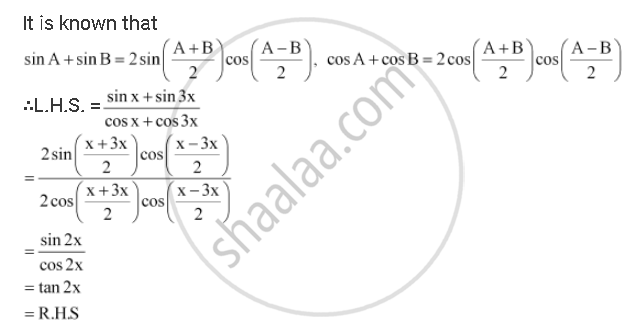CBSE (Arts) Class 11CBSE
Share

# Prove That (Sin X + Sin 3x)/(Cos X + Cos 3x) = Tan 2x - CBSE (Arts) Class 11 - Mathematics

ConceptTrigonometric Functions of Sum and Difference of Two Angles

#### Question

Prove that  (sin x + sin 3x)/(cos x + cos 3x) = tan 2x

#### SolutionIs there an error in this question or solution?

#### APPEARS IN

NCERT Solution for Mathematics Textbook for Class 11 (2018 to Current)
Chapter 3: Trigonometric Functions
Q: 19 | Page no. 73

#### Video TutorialsVIEW ALL 

Solution Prove That (Sin X + Sin 3x)/(Cos X + Cos 3x) = Tan 2x Concept: Trigonometric Functions of Sum and Difference of Two Angles.
S